Next: Example 10.4: The RC Up: Inductance Previous: Example 10.2: Energy density

## Example 10.3: The RL circuit

Question: A coil has a resistance of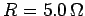and an inductance of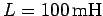. At a particular instant in time after a battery is connected across the coil, the current is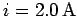, and is increasing at a rate of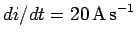. What is the voltageof the battery? What is the time-constant of the circuit? What is the final value of the current?

Answer: Application of Ohm's law around the circuit gives [see Eq. (257)]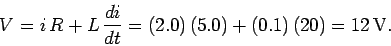The time-constant of the circuit is simply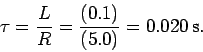The final steady-state currentis given by Ohm's law, with the inductor acting like a conducting wire, so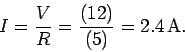Richard Fitzpatrick 2007-07-14